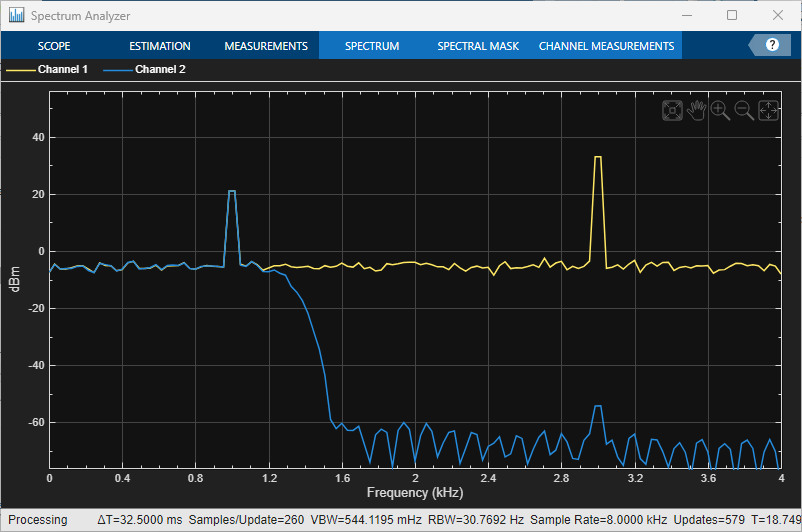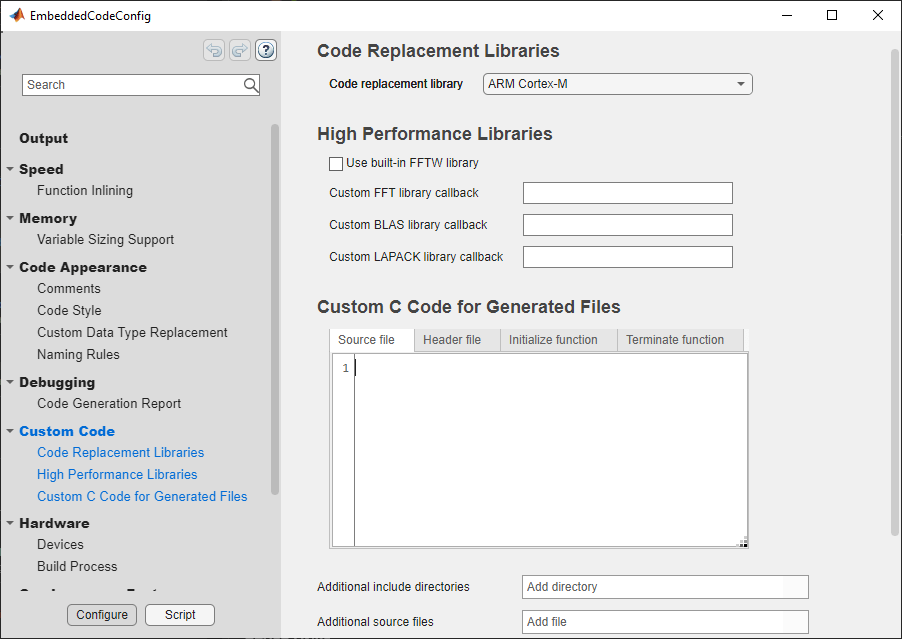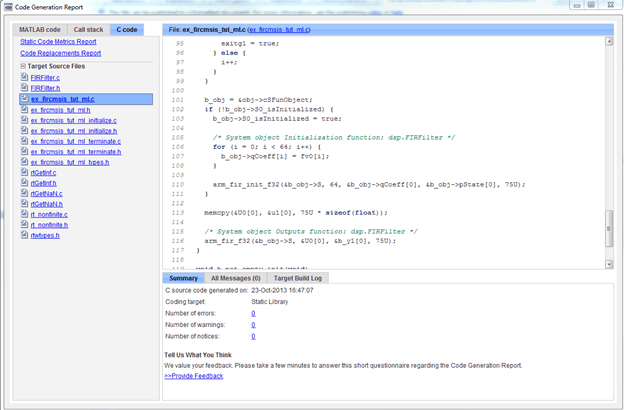# Verify FIR Filter on ARM Cortex-M Processor in MATLAB

This example shows how to use the Code Replacement Library (CRL) for ARM® Cortex®-M processor with DSP System object™. The example uses a dsp.FIRFilter System object to filter two sine waves of different frequencies.

### Prerequisites

• MATLAB Coder™

### Task 1: Setup and Simulate

1. Open the ex_fircmsis_tut_ml example function, which implements a lowpass FIR filter object.

2. Create two sine wave signals with 1KHz and 3KHz frequency, respectively.

```sin1 = dsp.SineWave('Amplitude',1,'Frequency',1000,... 'SampleRate',8000, 'SamplesPerFrame', 75,... 'OutputDataType', 'single'); ```
```sin2 = dsp.SineWave('Amplitude',4,'Frequency',3000,... 'SampleRate',8000, 'SamplesPerFrame', 75,... 'OutputDataType', 'single'); ```

3. Create a spectrum analyzer to view the spectrum of the input and filtered output.

```scope = spectrumAnalyzer('SampleRate',8e3,'ShowLegend',true,... 'PlotAsTwoSidedSpectrum', false, ... 'RBWSource', 'Property',... 'RBW',8000/260, 'Window','Kaiser', ... 'OverlapPercent', 80,... 'YLimits', [-76 56], 'SpectralAverages',10); ```

4. Simulate the example

```NN = 2000; for k = 1:NN x1k = sin1(); % generate 1K Hz sine wave x3k = sin2(); % generate 3K Hz sine wave n1 = randn(size(x1k), 'single')*sqrt(.05); % generate noise signal u1 = x1k+x3k+n1; y1 = ex_fircmsis_tut_ml(u1); scope([u1,y1]); end ```Once you are satisfied with simulation results with test inputs, you can go ahead to generate code for ex_fircmsis_tut_ml example function to able run with you ARM Cortex-M based hardware.

### Task 2: Configure for Code Replacement

1. Create a code generation configuration object for use with codegen when generating a C/C++ static library.

```cfgEx = coder.config('lib'); cfgEx.CodeReplacementLibrary = 'ARM Cortex-M'; cfgEx.HardwareImplementation.ProdHWDeviceType = 'ARM Compatible->ARM Cortex'; cfgEx.GenCodeOnly = true; ```

2. Open the Custom code panel of the configuration dialog and verify the settings.

```cfgEx.dialog ```1. Generate C code for the MATLAB function ex_fircmsis_tut_ml.m.

`codegen ex_fircmsis_tut_ml -args single(u1) -config cfgEx -report`

You can specify an output directory with option -d as described in codegen documentation to generate code in separate folder for each individual MATLAB function.

2. When code generation finishes successfully, click View report to display the code generation report.

3. Click on the ex_fircmsis_tut_ml.c file. Notice the CMSIS functions, arm_fir_init_f32 and arm_fir_f32 in the ex_fircmsis_tut_ml C function.### Task 4: Verify the Generated C Code on Target

The generated C code can be compiled and executed on ARM Cortex-M target by using a user-selected tool chain, for example, ARM® KEIL™ uVision® IDE.

### Task 5: Fixed-Point FIR Filter

Following similar steps of Task 3, you can generate fixed-point C code for the MATLAB function ex_fircmsis_tut_ml_q15.m.

```codegen ex_fircmsis_tut_ml_q15 -args fi(u1, true, 16, 15) -config cfgEx -report ```

### Task 6: Use Processor in the Loop (PIL)

Follow the steps in example Processor-in-the-Loop Verification of MATLAB Functions to perform verification using PIL.